# Understand Python Lambda Functions With Examples

In this tutorial, you will learn what lambda functions are in Python with the help of several examples. Know the pros and cons of using lambda functions.

Lambda functions in Python are anonymous functions, i.e., functions without names. Normal function can be defined using the def keyword, and the lambda keyword is used to define the lambda function. The Lambda function accepts any number of arguments but only a single expression.

Syntax: `lambda arguments : expression`

Below is a simple program that uses a lambda function.

Code:

```lowercaseText = 'python interpreter'

# lambda function that converts lowercase text to upper case
uppercaseText = lambda word : word.upper()

print(uppercaseText(lowercaseText))```

Output: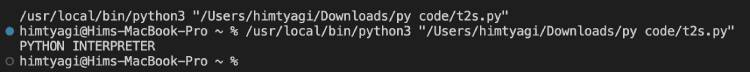Explanation: The lambda function uppercaseText converts the given string of lower case letters into upper case and returns the new string.

## Differences between Lambda function and normal function

 Lambda Function Normal Function 1 You can define lambda functions using the lambda keyword. On the other hand, you can define normal functions in Python using the def keyword. 2 Lambda functions are best suited for cases where you need to conduct small operations. Normal functions are best suited for cases where you need to write multiple lines of code. 3 One of the main downsides of using lambda functions in Python is that it reduces the readability of the code. You can maintain code readability by adding comments between statements using normal functions.

Below is the simple program that uses lambda and normal functions, which behave the same as others, i.e., the same functionality/logic.

Code:

```# normal function to add given three numbers
def sumfunc (num1, num2, num3):
return num1+num2+num3

# lambda function to add given three numbers
sumlamfunc = lambda n1, n2, n3 : n1 + n2 + n3

print('Total sum -',sumfunc(10, 20, 30))
print('Total sum -',sumlamfunc(10, 20, 30))```

Output: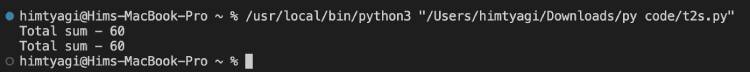Explanation: Here, the normal function (sumfunc) and lambda function (sumlamfunc) serve the same functionality, i.e., adding the given three numbers.

## Python Lambda Functions Examples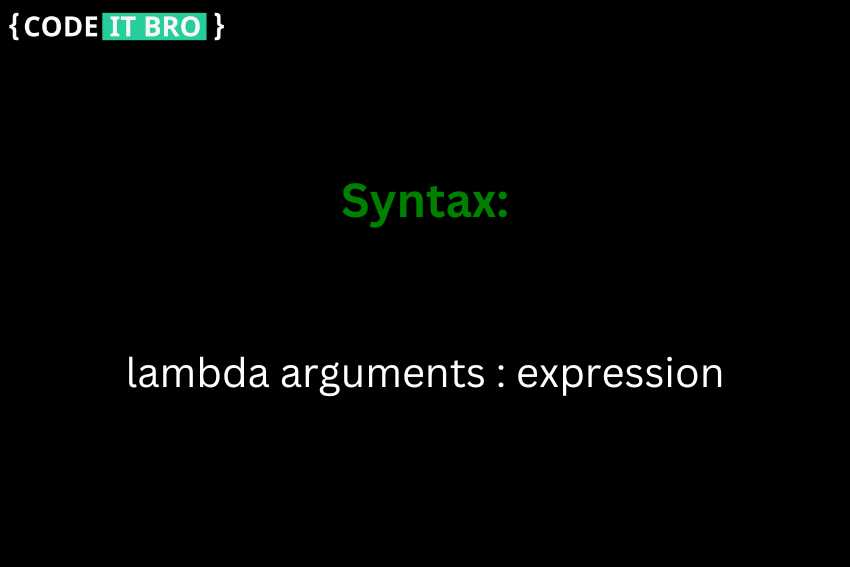Below are a few example programs where lambda functions are used in various cases

## Example 1: Lambda function with if else statements

Code:

```# lambda function that finds bigger number between given 2 numbers
Biggernumber = lambda num1, num2 : num1 if(num1 > num2) else num2

print('Bigger number between given 2 numbers is',Biggernumber(10,15))```

Output: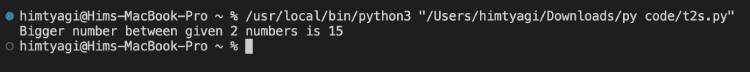Explanation: The lambda function – Biggernumber finds the larger number between the given two numbers and returns the largest number. Among 10 & 15, 15 is larger and is returned as a result.

Also ReadHow To Create a Python Web Server

## Example 2: Lambda function with list comprehension

Code:

```# lambda function that creates a list of numbers
numbers = [lambda n=i : n for i in range(1,10)]

# print the list of numbers formed from the lambda function
for num in numbers:
print(num())```

Output: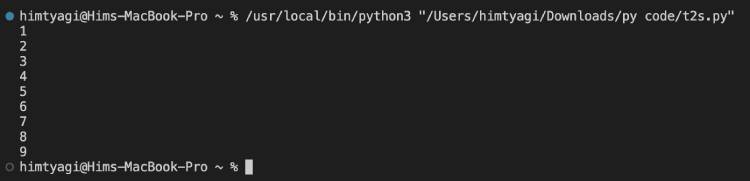Explanation: The above code creates a list of numbers using the lambda function.

Also Read15 Most Common Python Array Programs

## Example 3: Lambda function with filter() function

The filter() function accepts a function and a list of numbers. This filters out all the elements of a given sequence (list) for which the function returns True.

Code:

```numbers = [1,2,3,4,5,6,7,8,9,10]

# filters the even numbers from given set of numbers using lambda function
even_numbers = list(filter(lambda n : n%2==0, numbers))
print(even_numbers)```

Output: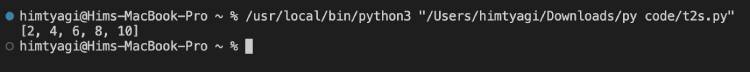Explanation: The above code creates a new list of even numbers using the lambda function from the given list of numbers.

## Example 4: Lambda function with map() function

The map() function accepts a function and list of numbers and returns a new list of numbers which consists of all the lambda-modified items returned by the function for each item.

Code:

```numbers = [1,2,3,4,5,6,7,8,9,10]

# finds the squares of numbers in the list
square_of_numbers = list(map(lambda n : n*n, numbers))
print(square_of_numbers)```

Output: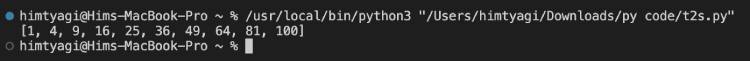Explanation: The above code returns a new list of numbers which are square values of a given list of numbers.

## Example 5: Lambda function with reduce() function

The reduce() function accepts a function and list of numbers and returns a reduced result. It is present in the functools module and can be imported. The reduce() method performs a repetitive operation over the pairs of the iterable.

Code:

```import functools
numbers = [10,2,30,4,50,6]

# finds the smallest number in the given list
smallest_number = functools.reduce(lambda n1,n2 : n1 if n1<n2 else n2, numbers)
print(smallest_number)```

Output: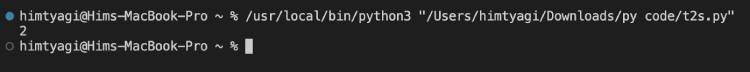Explanation: The above code returns the smallest number from the list using the lambda function passed into reduce() method.

## Pros and cons of using lambda functions in Python

Pros:

• Lambda functions are concise and easy to write since they are defined in a single line of code.
• You can use them to write code that is more functional in style, which can be more readable and maintainable in some cases.
• They are often used as arguments for higher-order functions, allowing you to write more expressive code by composing simple functions.

Cons:

• Because lambda functions are anonymous, it can be harder to debug code that uses them since there is no function name to look for in tracebacks.
• They are limited to a single expression, so they are unsuitable for writing larger or more complex functions.
• They do not support the return statement, so they may not be the best choice for functions that need to return a value.

Other Python Tutorials:

Scroll to Top